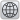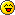BVE WorldWide
Would you like to react to this message? Create an account in a few clicks or log in to continue.

# Shear Command

3 posters##Shear Command

I am having problem while using the Shear command in csv route because I simply cannot understand the manual.
I have problem figuring out how the d, s, r parameters and normals work. Also the picture there is quite misleading because some of my friends have been complaining that they thought this command would actually cut the faces. I know what Shear means but I cannot understand what does d and s actually do as a normal and how to specific a normal. Also, since d and s don't have to be perpendicular, why is r based on a right triangle snd how does it actually work?
By the way there is a small mistake in the contents. SetCoordinates is there instead of SetTextureCoordinates. Is it an alias?
Also, Thanks Quork for correcting my mistake. Really sorry for that.zbx1425

Posts : 137
Join date : 2017-08-18
Location : China##Re: Shear Command

Code:
`private static void ApplyShear(MeshBuilder Builder, double dx, double dy, double dz, double sx, double sy, double sz, double r) {            for (int j = 0; j < Builder.Vertices.Length; j++) {                double n = r * (dx * Builder.Vertices[j].Coordinates.X + dy * Builder.Vertices[j].Coordinates.Y + dz * Builder.Vertices[j].Coordinates.Z);                Builder.Vertices[j].Coordinates.X += sx * n;                Builder.Vertices[j].Coordinates.Y += sy * n;                Builder.Vertices[j].Coordinates.Z += sz * n;            }            for (int j = 0; j < Builder.Faces.Length; j++) {                for (int k = 0; k < Builder.Faces[j].Vertices.Length; k++) {                    if (Builder.Faces[j].Vertices[k].Normal.X != 0.0f | Builder.Faces[j].Vertices[k].Normal.Y != 0.0f | Builder.Faces[j].Vertices[k].Normal.Z != 0.0f) {                        double nx = (double)Builder.Faces[j].Vertices[k].Normal.X;                        double ny = (double)Builder.Faces[j].Vertices[k].Normal.Y;                        double nz = (double)Builder.Faces[j].Vertices[k].Normal.Z;                        double n = r * (sx * nx + sy * ny + sz * nz);                        nx -= dx * n;                        ny -= dy * n;                        nz -= dz * n;                        World.Normalize(ref nx, ref ny, ref nz);                        Builder.Faces[j].Vertices[k].Normal.X = (float)nx;                        Builder.Faces[j].Vertices[k].Normal.Y = (float)ny;                        Builder.Faces[j].Vertices[k].Normal.Z = (float)nz;                    }                }            }}`

The code which applies shear is above.
Shear mapping (essentially) moves a vertex within 3D space proportionally to it's original relative position.

DX, DY & DZ represent the first vector.
SX, SY & SZ represent the second vector.

Essentially speaking, we define our fixed geometric plane with the first two vectors, and then shear around the third.
Imagine you spin your object so that the plane (rectangle) defined by D and S directly faces the camera.
Now chop this object into 2D by temporarily discarding the depth values.

R then represents the amount of shear to be applied. This is worked out as a proportion of our distance from 0,0,0
Quite why a R value of 1 represents 45 degrees of shear I'm not actually sure off the top of my head, it's probably got a mathematical significance somewhere, but would have to think hard about that oneShear will be applied to any normals defined in the face exactly as per the verticies. If no normals are defined, it'll work out the normals based upon the final face.

##Re: Shear Command

leezer3 wrote:
Imagine you spin your object so that the plane (rectangle) defined by D and S directly faces the camera.
Now chop this object into 2D by temporarily discarding the depth values.

R then represents the amount of shear to be applied. This is worked out as a proportion of our distance from 0,0,0.
That was a lively explanation. Thanks.
But I still didn't understand some of it. As the object is "chopped" on the surface defined by D and S, then each face is seen as an 2d face and did a shear transform(while edges parallel to S remains same and others being alternated), and their depth value remain unaffected, is that right?
Also, are S and D required to be perpendicular? What will happen if they are not?zbx1425

Posts : 137
Join date : 2017-08-18
Location : China##Re: Shear Command

Yeah, I think that's essentially right.
3D math makes my head hurt mindIn any case, S and D are not required to be perpendicular, they just define the plane.

##Re: Shear Command

Reminds me of quadratic equations, which I never fully understood and I forgot long ago!!graymac

Posts : 2127
Join date : 2011-08-28
Location : Co Mayo, Eire##Re: Shear Command